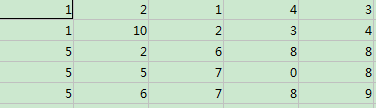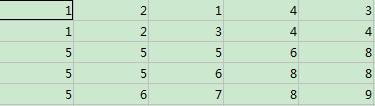# 中值滤波 matlab程序实现（一）

clc
close all
f=[1 2 1 4 3;
1 10 2 3 4;
5 2 6 8 8;
5 5 7 0 8 ;
5 6 7 8 9];
[row, col]=size(f);
k=f;
for r=2:row-1
for c=2:col-1
m=ones(3, 3);
n=ones(3, 3);
b=k;
n(2,2)=b(r,c);    %22
n(1,1)=b(r-1,c-1);%11
n(1,2)=b(r-1,c);  %12
n(1,3)=b(r-1,c+1);%13
n(2,1)=b(r,c-1);  %21
n(3,1)=b(r+1,c-1);%31
n(3,2)=b(r+1,c);  %32
n(2,3)=b(r,c+1);  %23
n(3,3)=b(r+1,c+1); %33
out=n.*m;
mid=median(out(:));
f(r,c)=mid;
end
end%%%median函数能取一列或者一行数的中值

12-1510-06
04-15156
01-081935
07-104万+
02-164万+
11-192万+
04-16
10-305232
07-051395
05-12
11-28285
12-19
05-06
12-02
01-047万+
©️2020 CSDN 皮肤主题: 大白 设计师:CSDN官方博客